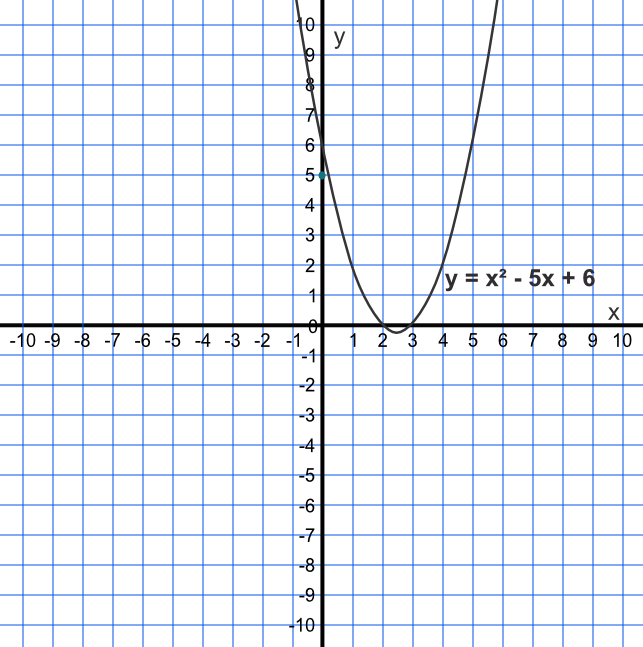Roots

## Roots

The roots of the function are found when y = 0 (that is, the curve crosses the x-axis).

A quadratic may cross the x-axis twice, or it may only touch the x-axis, or it may not cross the x-axis at all. In the first instance, the quadratic will have two roots; in the second instance there will be one root (actually the same root repeated); and in the third instance no real roots (the graph does not cross y = 0).

The values of x when it crosses the x-axis are the solutions to the equation.

## Example 1

By drawing a graph, estimate the roots for y = x^2 - 5x + 6.

 x -1 0 1 2 3 4 5 6 x^2 - 5x + 6 12 6 2 0 0 2 6 12

Answer: x=2 and x=3## Example 2

With the two roots from the answer, above, show that they are valid roots.

The roots are given where the line crosses the x-axis, at (2,0) and (3,0)

When x=2 or x=3 then y=0